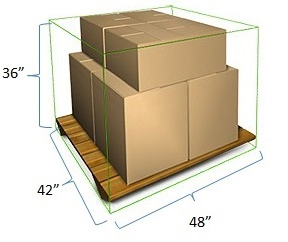Home > Tools & Forms > Density Calculator

## Density Calculator

Calculate Density by:
1
=

=

a. Required field
b. Required field when total handling weight is not entered
c. Required field when unit weight is not entered

The results provided are based on the information entered and may differ based on actual shipment characteristics

#### How to Measure a Shipment and Determine Handling Unit Density

1. Measure dimensions of handling unit in inches at its greatest distance

Note: In the case where it's a palletized handling unit, the greater of the dimensions of the pallet or the dimensions of the freight will be used to determine the length and width

2. Multiply the dimensions (L x W x H) to determine the total cubic inches of the handling unit

3. Divide the total cubic inches by 1728 to convert to total cubic feet

4. Divide the total handling unit weight (including packaging and pallet weight) by the total cubic feet to determine the handling unit density